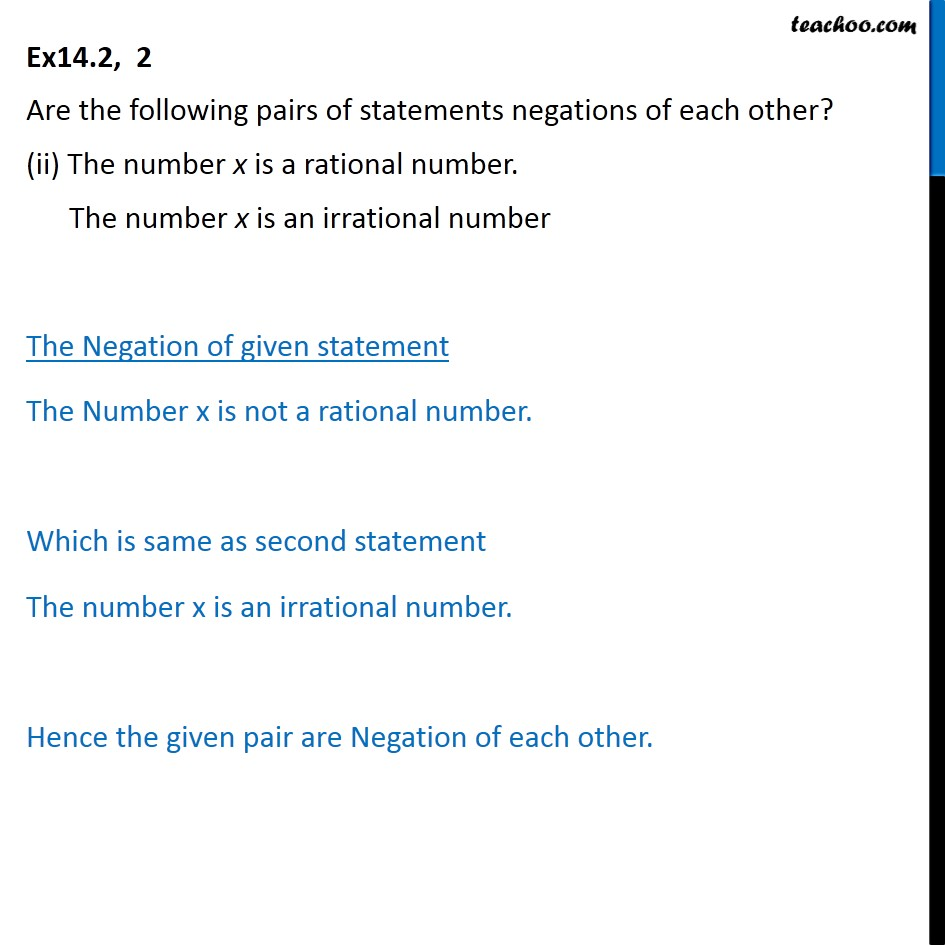Negation of Statements

Mathematical Reasoning
Serial order wiseLearn in your speed, with individual attention - Teachoo Maths 1-on-1 Class

### Transcript

Ex14.2, 2 Are the following pairs of statements negations of each other? (ii) The number x is a rational number. The number x is an irrational number The Negation of given statement The Number x is not a rational number. Which is same as second statement The number x is an irrational number. Hence the given pair are Negation of each other.